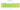# ރ

## Maldivian rufiyaa

#### how has the value of the currency changed in the last year?## USD 1 = MVR 15.445

How much is10 US dollars worth inMaldivian rufiyaas?
At the current exchange rate, 10 US dollars is worth 154.45 Maldivian rufiyaas
How much is50 US dollars worth inMaldivian rufiyaas?
At the current exchange rate, 50 US dollars is worth 772.25 Maldivian rufiyaas
How much is100 US dollars worth inMaldivian rufiyaas?
At the current exchange rate, 100 US dollars is worth 1,544.5 Maldivian rufiyaas
How much is500 US dollars worth inMaldivian rufiyaas?
At the current exchange rate, 500 US dollars is worth 7,722.5 Maldivian rufiyaas
How much is2,000 US dollars worth inMaldivian rufiyaas?
At the current exchange rate, 2,000 US dollars is worth 30,890 Maldivian rufiyaas
How much is10 US dollars worth inMaldivian rufiyaas?
At the current exchange rate, 10 US dollars is worth 154.45 Maldivian rufiyaas
How much is50 US dollars worth inMaldivian rufiyaas?
At the current exchange rate, 50 US dollars is worth 772.25 Maldivian rufiyaas
How much is100 US dollars worth inMaldivian rufiyaas?
At the current exchange rate, 100 US dollars is worth 1,544.5 Maldivian rufiyaas
How much is500 US dollars worth inMaldivian rufiyaas?
At the current exchange rate, 500 US dollars is worth 7,722.5 Maldivian rufiyaas
How much is2,000 US dollars worth inMaldivian rufiyaas?
At the current exchange rate, 2,000 US dollars is worth 30,890 Maldivian rufiyaas